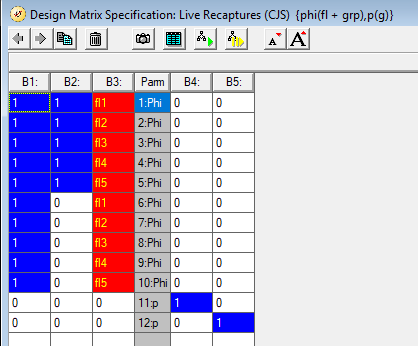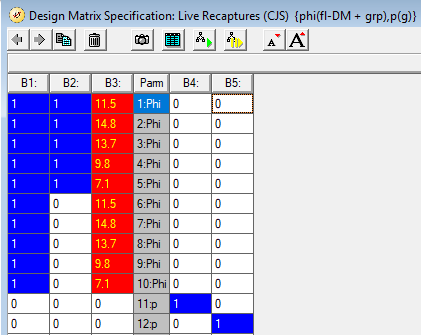For your homework for this week, please complete the following by the beginning of class next Thursday. I ask that you try to do the homework by next Tuesday but will answer questions on the homework on Tuesday so you can turn it in on Thursday.

Data set

The file “hw03_data.inp” contains information from a live-recaptures study of 2 species of fish collected annually over 6 years. Species identity is provided in the input file as groups: species A is group 1, and species B is group 2. Information on river flow and turbidity for years 1 through 5 is provided as individual covariates in 10 different columns: fl1, fl2, …, fl5, and turb1, turb2, …, turb5. The flow values for years 1-5 are 11.5, 14.8, 13.7, 9.8, and 7.1. The turbidity values are 2.9, 4.3, 2.8, 4.4, and 1.1.

Setting up the problem in Program MARK

When you set the problem up in MARK, you need to tell it that you have data for 2 groups (sppA and sppB) and that there are 10 individual covariates in the data set (fl1, fl2, …, fl5, and turb1, turb2, …, turb5). If you name the groups and the covariates when you set the problem up, it will be easier to work with the output, so please take the time to do that.

1. How many PIMs are there for this problem?

2. How many columns are there in each of the PIMs?

Models to evaluate

For $$\phi$$, please run 5 base models: $$\phi_.$$, $$\phi_t$$, $$\phi_{flow}$$, $$\phi_{turb}$$, and $$\phi_{flow + turb}$$. Also, add group to each of those models, e.g., $$\phi_.$$ becomes $$\phi_g$$; $$\phi_t$$ becomes $$\phi_{t+g}$$; $$\phi_{flow}$$ becomes $$\phi_{flow+g}$$, etc.. This yields 10 models for $$\phi$$. For each of those 10 models, evaluate 2 versions: 1 with $$p_.$$ and 1 with $$p_g$$ such that you evaluate 20 models. Finally, add a 21st model that is $$\phi_{g*t},p_g$$.

I recommend numbering the PIMs for $$\phi$$ as you would for $$\phi_{g*t}$$ and the PIMs for $$p$$ as you would for $$p_g$$. There are lots of other ways to do it, however, so do as you see fit. For example, you can use the pre-defined models to achieve some of the models above. But, working with 1 set of PIM numbering and then constraining the parameters with the design matrix might help improve your understanding.

When you build the models that use flow and/or turbidity, you can enter information on the annual values in 2 different ways. You can either (1) enter the names of individual covariates into the design matrix, where the names refer to covariates provided in the input file (this is what you’ll do this week) or (2) enter the value of the annual covariate into the design matrix. These alternatives are presented in Chapter 11 of CW on pages 11-30 through 11-33. Here, because the values are stored in the input file, you can use the names. This makes your input file quite a bit bigger (10 additional columns), which might seem undesirable given that all individuals have the exact same set of values for the 10 covariates and because MARK allows you to simply enter the values directly into the design matrix. But, as you’ll see below, there are some advantages for obtaining certain plots if you work with the stored values. Below is an example of the design matrix for model $$\phi_{flow + group}, p_g$$ for PIMs numbered as I recommend above.1. Provide an AIC table that is ordered by AICc (lowest at the top). You can obtain a copy using the “Output” menu when the Results Browser is active. If you output the table to Excel, you can work on it in Excel pretty efficiently to prepare it for copying to WORD, printing, etc. E.g., you can delete the time-stamp column, format the data portion as numbers with 2 decimal places, change column widths, etc.

2. Based on the AIC table in the results browser, which model structure(s) was(were) most supported by the data and what general statements can you make about evidence for variation in $$\phi$$ and $$p$$ among years and groups?

3. For the top model, what are the $$\hat\beta_i$$ and how would they be used to obtain the values for $$\hat\phi_{g,t}$$ and $$\hat{p}_g$$.

4. Write out the equation for obtaining an estimate of $$\hat\phi_2$$ for species A and for species B.

5. Which model has the lowest deviance, i.e., best fit, and why is this so?

6. Which model has the highest deviance, i.e., worst fit, and why is this so?

7. In the best-supported model that contains both flow and turbidity, how do the values for $$\hat\beta$$ and $$\widehat{SE}(\hat\beta)$$ compare for flow and turbidity. Given this, which covariate appears to be more strongly associated with annual variation in $$\phi$$?

8. Highlight the top model in the Results Browser and ask for an individual covariate plot for $$\hat\phi_{spp.A}$$. You can do this using the 8th icon in from the left on the Results Browser window or from the Output menu, under the sub-list for Specific Model Output. On the covariate plot menu, work with the first parameter (“1:Phi”) and covariate “fl1”, which were used together in the design matrix. Use a minimum flow value of 7 and maximum value of 15 (~range of values), check the box for sending the estimates to Excel, and hit OK to view the plot, which can be saved. How much does survival vary over the range of flow values and how precise are the estimates?

9. Highlight the top model in the Results Browser and ask for a line graph for $$\hat\phi_{spp.A}$$. You can do this using the 7th icon in from the left on the Results Browser window. Plot parameters “1:Phi” through “5:Phi”. Why does this look so different from the plot you created for the last question? Use the “Add Data Series” menu on the plot window to add a line graph for the 2nd species, i.e., plot parameters “6:Phi” through “10:Phi”. What inferences can you make from this plot?

Note: you could also achieve the model $$\phi_{flow + group}, p_g$$ using the design matrix below. This is fine and gives you the same estimates for apparent survival in each year and the same estimate coefficients for the model as you obtained by using the individual-covariates approach. But, you cannot use MARK to make the individual covariate plot with this approach. You could still obtain the line graph of this model’s annual estimates of parameter values by year.